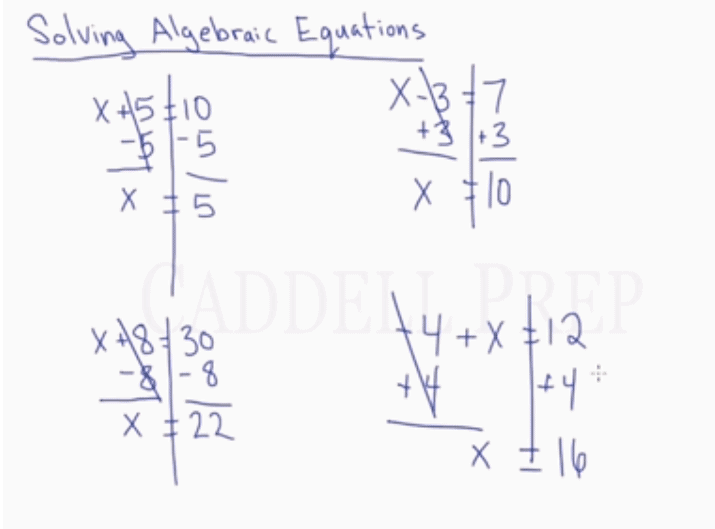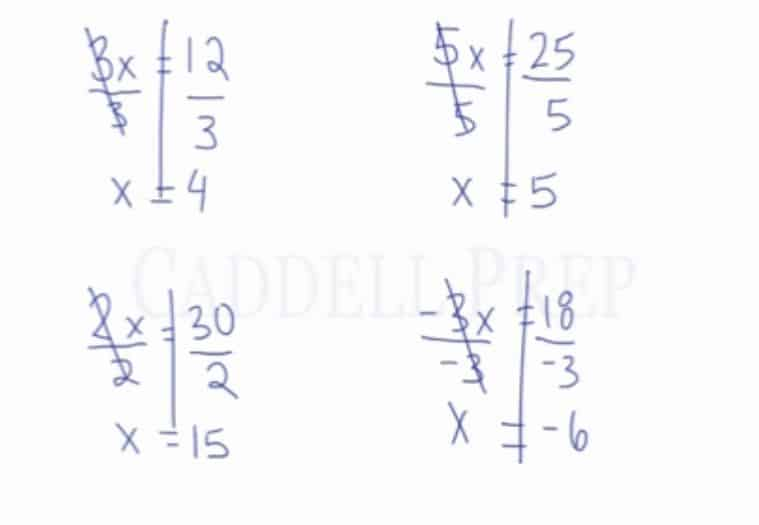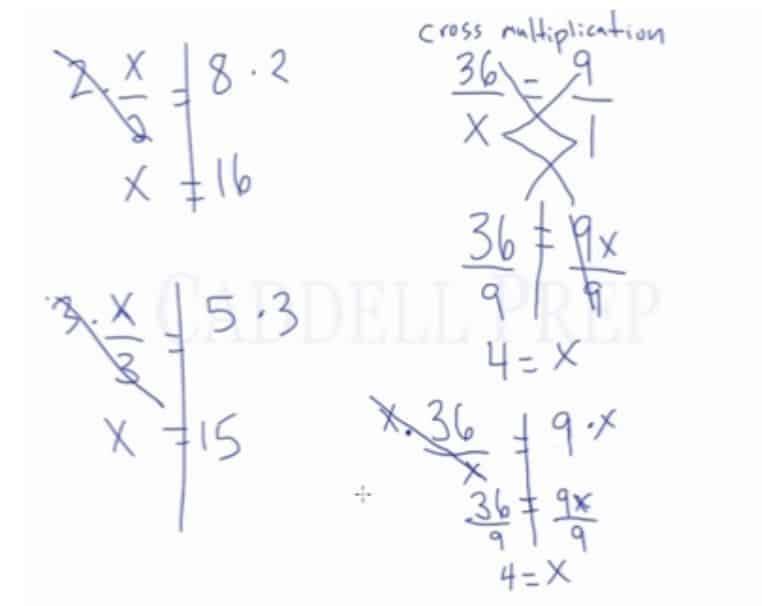In this video, students will be learning how to solve algebraic equations using inverse operations. After you finish this lesson, view all of our Pre-Algebra and Algebra 1 lessons and practice problems.

For example:$36 = 9x$

To solve for$x$, we will use inverse operations.

Since$x$ is being multiplied by 9, the inverse operation would be to divide both sides by 9.$\frac{36}{9}=\frac{9x}{9} \leftarrow$ Divide by 9 on both sides$4 = x$

## Examples of Solving Algebraic Equations (One-Step)

### Examples 1$x+5=30$

We have to eliminate$5$ by subtracting$5$ on both sides

Therefore,$x+5-5=30-5$

Now, we have:$x=25$

### Examples 2$8x=56$

To solve for$x$, we will use inverse operations.

Since$x$ is being multiplied by$8$, the inverse operation would be to divide both sides by$8$$\dfrac{8x}{x}=\dfrac{56}{8}$

Now, we have:$x=7$

## Video-Lesson Transcript

In this video, students will be learning how to solve algebraic equations using inverse operations.

### Solving Equations that Have Addition or Subtraction

Let’s start off with some simple ones.$x + 5 = 10$

To get the value of$x$ we have to subtract$5$ from both sides of the equation.

It may be useful to draw a long vertical line through the equal sign. For you to remember that whatever you do on one side, you have to do on the other side, too.

The answer is$x = 5$.

Let’s have more examples.$x + 8 = 30$

We have to subtract$8$ from both sides.

And we will come up with$x = 22$.

Here, we have$x - 3 = 7$.

To get rid of$- 3$ we have to add$3$ on both sides.

Now we have$x = 10$.

Also, let’s do${-4} + x = 12$.

To get rid of${-4}$ we should add$4$.

So we’ll have$x = 16$.### Solving Equations that Have Multiplication or Division

Let’s now look at some examples involving multiplication.

So for example, we have$3x = 12$. This means$3 \times x = 12$.

To do this, we have to identify that the inverse of multiplication is division.

So we have to divide both sides by$3$.

We will come up with$x = 4$.

More example:$2x = 30$

Then divide both sides by$2$

The answer is$x = 15$

Let’s have$5x = 25$ then divide both sides by$5$ to get$x = 5$.

Another example is${-3x} = 18$.

Don’t be tricked by the negative sign. It is not a subtraction sign.

So to get rid of${-3}$ we just have to divide both sides by${-3}$.

And the answer is$x = {-6}$Now, let’s have examples involving division.$\dfrac{x}{2} = 8$

So we have to do the inverse of division which is multiplication. Let’s multiply both sides by$2$.

And we get$x = 16$.

Let’s also try$\dfrac{x}{3} = 5$. Then multiply both sides by$3$. We’ll have$x = 15$.

Here’s another method to solve equations involving division. You may have seen it before. It is called ‘cross-multiplication’.

We have$\dfrac{36}{x} = 9$

To do cross-multiplication, it’s important to show both sides of the equation as fractions. So we should have$\dfrac{36}{x} = \dfrac{9}{1}$.

What we’re going to do is$36 \times 1 = 36$.

Then$9 \times x = 9x$.

We have$36 = 9x$.

We still want to isolate$x$ so we’ll divide both sides by$9$.

The answer is$4 = x$.

### Solving Equations that Involve Cross-Multiplication

Now let’s solve it without cross-multiplication.$\dfrac{36}{x} = 9$

Let’s multiply both sides by$x$ and we have$36 = 9x$.

This is similar to the one above.

So let’s divide both sides by$9$ and we still find that$4 = x$.To sum up, for simple algebraic equations, we just can do inverse operations to get the value of$x$.